# Exploring Breadth First Search and Depth First Search in a graph

You might have encountered the words, breadth and depth in real-world scenarios. Breadth implies the complete range of knowledge of any given subject or topic. On the other hand, depth in terms of learning touches on the degree to which a particular subject is magnified or explored. Let’s begin with the breadth first search or the BFS of a given graph. Now BFS does not refer to Best Friends from school but Breadth-First Search.

Exploring Breadth First Search or Breadth First Traversal

BFS is an algorithm that is designed to search for a graph or tree data formation. It usually travels in a breadthward motion and utilises a queue as a prompt to identify the next vertex to commence a traversal. If a roadblock is encountered or no adjacent node is found, the tree root or the source node is removed for the queue. The traversal of the graph usually begins with a ‘search key’ or the initialising node. Imagine a hotel with so many floors and rooms as nodes, a breadth-first traversal algorithm is like a cleaning staff that will clean rooms floor by floor. All neighbouring nodes at the current depth or floor with the example above will be visited to clean before moving to the vertices or rooms on the next floor. No node is expected to be revisited as one would not expect hotel staff to clean the same room twice in the same period. Once a room is cleaned, it is ticked on a sheet as a visited while with BFS, the neighbouring reversed node is enqueued or marked as visited,

Rules are important to ensure procedures are followed or order is maintained. There are relevant rules for a BFS algorithm.

• Rule Number One: From the tree root or ‘search key’ visit the adjacent unvisited vertex. In some cases, if there are three neighbouring nodes, an alphabetic or serial logic can be applied to choose the nearest node. Once visited, the node is displayed and enqueued.
• Rule Number Two: In the event, a neighbouring or adjacent node is not available, the tree root or initial node is removed from the queue and previously visited node plays the role of a search key (tree root or source node).
• Rule Number Three: The final rule is simply a repetition of the above two rules until all nodes are visited.

Below is a graphical example of how breadth-first search unfolds

Step 1: The queue is initiated with node R as the tree root.

Queue: [ ]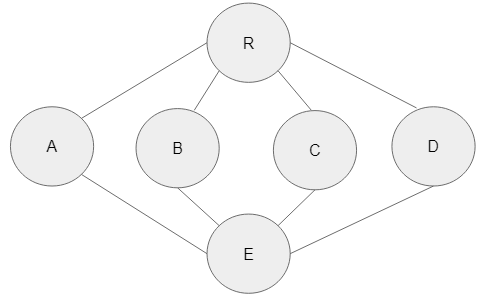Step 2: Graph traversal commences with the starting node R. The root node is now marked as visited.

Queue: [ ]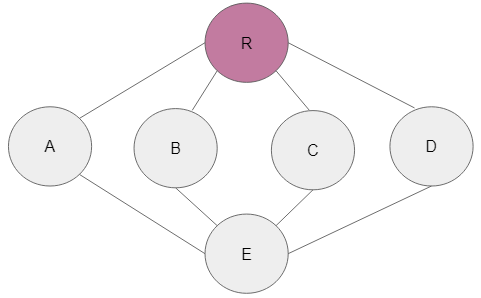Step 3: The neighbouring node of A is now next in line and subsequently marked as visited.

Queue: [ A ]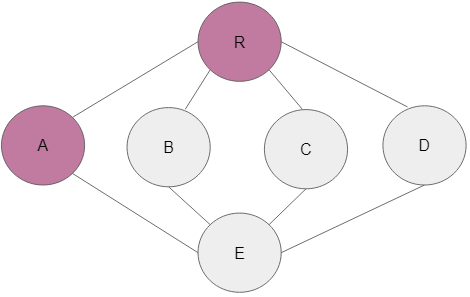Step 4: There are a couple of neighbouring nodes to A that can be added to the enqueued and marked. On this occasion, alphabetically, node B is the next logical neighbouring node that should be added to the queue and marked accordingly. That is what has been implemented in this case.

Queue: [B, A ]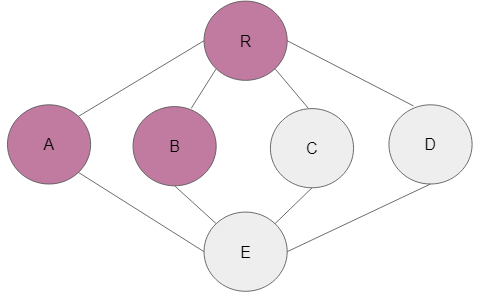Step 5: Node C is next in line and is visited and marked as well.

Queue: [C, B, A ]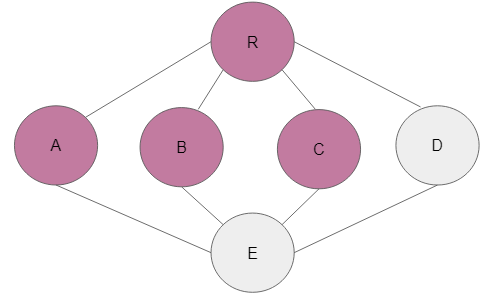Step 6: Node D is the adjacent node to C and alphabetically ticks the box.

Queue: [D, C, B, A ]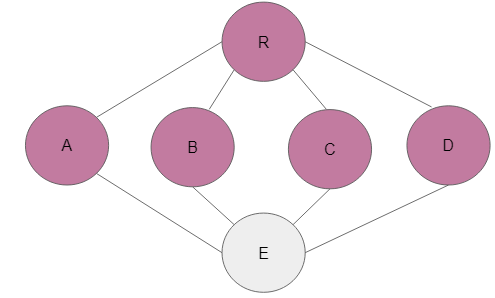Step 7: All adjacent nodes to R have all been visited. In this case, R will be dequeued and A will be the root node that leads to the enqueuing and further marking of E.

Queue: [ E, D, C, B]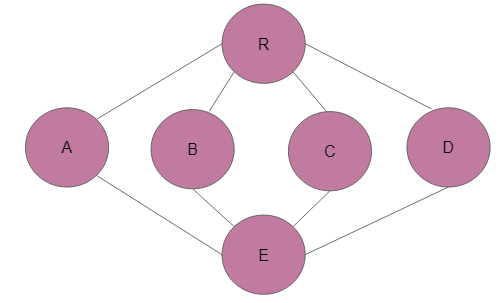Here is a Python code implementation of BFS with nodes assigned a numerical label as opposed to the strings in the diagram representation above.

``````from collections import defaultdict

# This class represents a directed graph
# using adjacency list representation
class Graph:

# Establising the constructor
def __init__(self):

self.graph = defaultdict(list)

def addEdge(self,n,r):
self.graph[n].append(r)

def BFS(self, t):

visited = [False] * (max(self.graph) + 1)

queue = []

queue.append(t)
visited[t] = True

while queue:

# Dequeue a vertex from
# queue and print it
t = queue.pop(0)
print (t, end = " ")

for i in self.graph[t]:
if visited[i] == False:
queue.append(i)
visited[i] = True

# Create a graph given in
# the above diagram
g = Graph()
g.addEdge(0, 1)
g.addEdge(0, 2)
g.addEdge(1, 2)
g.addEdge(1, 0)
g.addEdge(2, 0)
g.addEdge(2, 3)
g.addEdge(3, 3)

print("Executing BFS from (initialising from node 0)")
g.BFS(0)

#Output
Executing BFS from (initialising from node 0)
0 1 2 3 ``````

Examining Depth First Search or Depth First Traversal

Depth-first search traversal is different from breadth-first due to the direction of the search carried out. With depth-first search, the movement from a node to an adjacent one is depthward in nature. The depth-first search uses a stack to recall to get to the neighbouring unvisited vertex while breath-first uses a queue. A stack is the opposite of a queue, as the former is an ordered list of properties where all inclusions and exclusions are implemented at the same end. On the other hand, a queue has two sides, one end is used for inserted visited nodes and the other to delete data. The queue is usually referred to as FIFO (first-in, first-out) and the stack as the LIFO rule (last-in, first-out).

The below graph is an expression of how a depth-first traversal works. It does not search based on alphabetical order. The next node from a depthward motion is marked as visited and added to the stack. Node S is the tree root or the starting node and the traversal moves deep by visiting and adding nodes E, H and K to the stack. The edge labels indicate the order of the traversal with node G, becoming the last node in the traversal.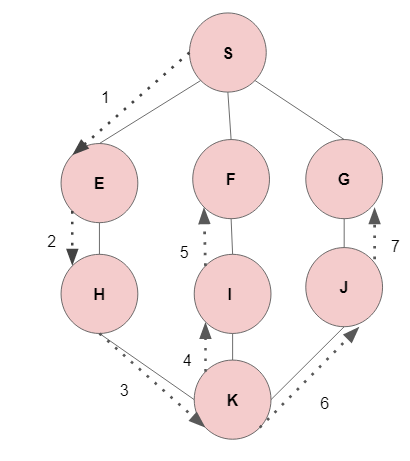To implement the above traversal, certain rules were pertinent. You could refer to them as the rules of depth-first search.

Below are the rules that should guide the implementation of DFS

Rule Number One: The adjacent unvisited node should be visited. It needs to be marked as visited and added to the DFS stack. The traversal highlights a depthward motion.

Rule Number Two: When all adjacent vertices from a given node are visited, the most recently added node is deleted from the stack to make way for a new insertion.

Rule Number Three: Rules one and two are repeated until all nodes have been visited and added to the stack.

Sequential implementation of DFS

Step 1: This is a network with an empty stack. Vertex R is the root node and the stack is being initialised.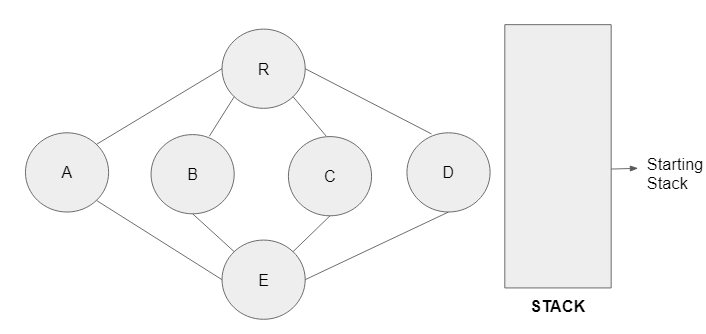Step 2: Node R is visited and added to the stack. It is now the top node in the stack and the adjacent node from a depthward motion will be executed in the next step.

Step 3: Node A is adjacent to R and is visited next in that order. It is added to the stack and becomes the top node.

Step 4: Node E, is visited and also added to the stack. In a breadth-first search, node B would have been the adjacent vertex. In this case, vertex E becomes the top as it is the most recently visited one.

Step 5: Node B is unvisited and adjacent to E. In this instance, B is visited and added to the stack as the top node.

Step 6: There are no unvisited adjacent nodes to B. The vertex B will now be removed from the stack and E assumes the role of the top node to aid a smooth continuation of the traversal process.

Step 6: Node C is now visited and added to the stack to become the top vertex.

Step 7: There are no unvisited neighbouring nodes to C. Node C is now removed from the stack and the previously visited E becomes the top node to allow for a clear traversal to an unvisited vertex.

Step 8: Node D was the only one not visited in the previous sequence or step. It is now visited and added to the stack as the top node.

Here is the python code implementation using the node labels above to print the DFS order.

``````from collections import defaultdict

class Graph:

def __init__(self):

# The default dictionary for the graph
self.graph = defaultdict(list)

# function to add an edge to graph
def addEdge(self, u, v):
self.graph[u].append(v)

# A DFS function
def DFSUtil(self, v, visited):

# Mark the current vertex as visited
# and print it
visited.add(v)
print(v, end=' ')

# Recur for all the vertices
# adjacent to this vertex
for neighbour in self.graph[v]:
if neighbour not in visited:
self.DFSUtil(neighbour, visited)

# recursive DFSUtil() for DFS traversal
def DFS(self, v):

# Create a set to store visited vertices
visited = set()

# Requesting the recursive helper function
# to print the DFS traversal graph
self.DFSUtil(v, visited)

# Creating a graph and connecting the nodes
g = Graph()
g.addEdge('S', 'E')
g.addEdge('E', 'H')
g.addEdge('H', 'K')
g.addEdge('K', 'I')
g.addEdge('I', 'F')
g.addEdge('K', 'J')
g.addEdge('J', 'G')

print("Executing DFS from (initialising from node E)")
g.DFS('E')``````